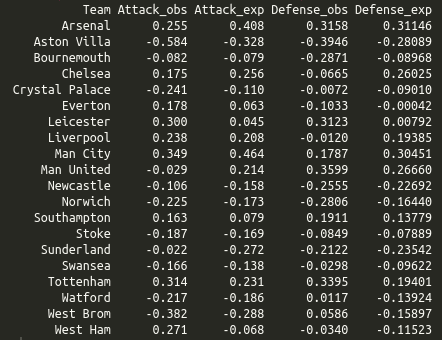Expected goals from bookmaker odds

I recently read an interesting paper called The Betting Odds Rating System: Using soccer forecasts to forecast soccer by Wunderlich and Memmert. In their paper they develop av variant of the good old Elo rating system. Instead of using the actual outcomes of each match to calculate the ratings, they use the probabilities of the outcomes, which they get from bookmaker odds.

I was wondering if a similar approach could be used together with the goalmodel package I released a couple of months ago. The models available in the package are models I have written about extensively on this blog, and they all work as follows: You use the number of goals scored to get some ratings of the goal scoring and goal conceding rates of each team. You then use these ratings to forecast the expected number of goals in the upcoming games. These expected goals can then be used to calculate the probabilities of the outcome (Home win, draw, away win). A crucial step in these calculations is the assumption that the number of goals scored follow the Poisson distribution (or some related distribution, like the Negative Binomial).

But can we turn this process the other way around, and use bookmaker odds (or odds from other sources) to get expected goals and maybe also attack and defense ratings like we do in the goalmodel package? I think this is possible. I have written a function in R that takes outcome probabilities and searches for a pair of expected goals that matches the probabilities. You can find it on github (Edit: The function is now included in the goalmodel package.). This function relies on using the Poisson distribution.

Next, I have expanded the functionality of the goalmodel package so that you can use expected goals for model fitting instead of just observed goals. This is possible by setting the model argument to “model = ‘gaussian'” or to “model = ‘ls'”. These two options are currently experimental, and are a bit unstable, so if you use them, make sure to check if the resulting parameter estimates make sense.

I used my implied package to convert bookmaker odds from the 2015-16 English Premier League into probabilities (using the power method), found the expected goals, and then fitted a goalmodel using the least squares method. Here are the resulting parameters, from both using the expected goals and observed goals:I wanted to use this season for comaprison as this was the season Leicester won unexpectedly, and in the Odds-Elo paper (figure 6) it seemed like the ratings based on the odds were more stable than the ones based on the actual results, which increased drastically during the season. In the attack and defense ratings from the goalmodels we see that Leicester have average ratings (which is what ratings close to 0 are) in the model based on odds, and much higher ratings based on the actual results. So the goalmodel and Elo ratings seem to agree, basically.

I also recently discovered another paper titled Combining historical data and bookmakers’odds in modelling football scores, that tries something similar as I have done here. They seem to do the same extraction of the expected goals from the bookmaker odds as I do, but they don’t provide the details. Instead of using the expected goals to fit a model, they fit a model based on actual scores (similar to what the goalmodel package do), and then they take a weighted average of the model based expected goals and the expected goals from the bookmaker odds.